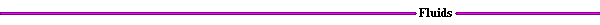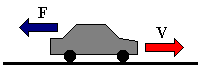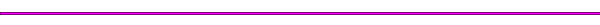Vehicle Power RequirementsHow much power does it take to go 60 mph? 80 mph?  The weight of the car and its speed all are factors that affect the amount of power required. The force balance of a vehicle is shown in Figure 4.Figure 4. Force Balance

The forces acting on the car are caused by internal, tire, and air resistance. The resultant of these forces, the total drag force, FD, can be estimated by the following equation:Where:

cR     =     coefficient of rolling resistance
cD      =     drag coefficient
m      =     mass of vehicle [kg]
A      =     frontal surface area [m2]
g       =     9.8 m/s
r       =     density of are, 1.2 kg/m3 @STP
The coefficients of rolling resistance and drag are determined from experiment. A typical value for the coefficient of rolling resistance is 0.015. The drag coefficient for cars varies, a value of 0.3 is commonly used.

The power output requirement can be determined from the drag force given above and the vehicle velocity.

P = FDV

Given the mass of a vehicle and its frontal surface area, a plot can be drawn showing the power requirements for a range of speeds. The Power Requirement Applet plots this relationship.

The power required to accelerate to a given speed is also of interest. More power is required for more acceleration. The Acceleration Applet compares the power required to accelerate from 0 to 60 mph for a range of times.

The basic definition for the acceleration force (neglecting drag !) is given by:

F = ma

Assuming that the force required to accelerate a vehicle from 0 to 60 mph can be determined from the above equation, then the power necessary to accelerate to a given velocity is:

P = maV

Where:

m     =    mass of the vehicle
a      =    acceleration = DV/DT
DV   =    60 - 0 = 60 mph = 26.82 m/s
V     =    final velocity, 60 mph